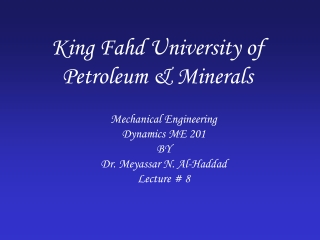DownloadDownload PresentationKing Fahd University of Petroleum & Minerals

King Fahd University of Petroleum & Minerals

Download PresentationKing Fahd University of Petroleum & Minerals

- - - - - - - - - - - - - - - - - - - - - - - - - - - E N D - - - - - - - - - - - - - - - - - - - - - - - - - - -
Presentation Transcript

1. King Fahd University of Petroleum & Minerals Mechanical Engineering Dynamics ME 201 BY Dr. Meyassar N. Al-Haddad Lecture # 8

2. 12.10 Relative-Motion Analysis of Two Particles Using Translating Axes Relative Motion (Translating Axes) - Consider two particles A and B. Both are moving with respect to fixed axes X-Y - The translating axes x-y is moving with particle B - The position vector of the particle A measured relative to the x-y axes is (A/B means A with respect to B)

3. KINEMATICS OF PARTICLES If is the absolute position of B, then Take derivative with respect to time : or and or

4. A B 160 km/h 180 km/h

5. y x EXAMPLE The passenger aircraft B is flying east with velocity vB = 800 km/h. A jet is traveling south with velocity vA = 1200 km/h. What velocity does A appear to a passenger in B ? Solution

6. EXAMPLE (Cont.) Absolute value : The direction of y West of South x

7. q Example

8. Example

9. Problem At the instant shown, the car at A is traveling at 10 m/s around the curve while increasing its speed at 2 m/s2. The car at B is traveling at 18.5 m/s along the straightway and increasing its speed at 5 m/s2. Determine the relative velocity and relative acceleration of A with respect to B at this instant

10. Thank you